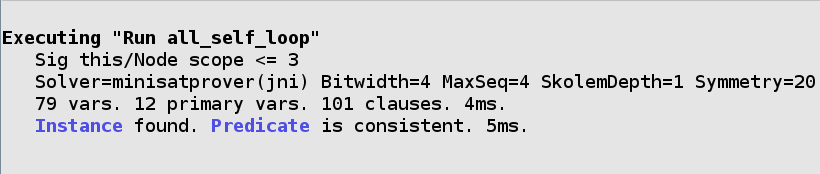# Commands¶

A command is what actually runs the analyzer. It can either find models that satisfy your specification, or counterexamples to given properties.

By default, the analyzer will run the top command in the file. A specific command can be run under the `Execute` menu option.

## `run`¶

run tells the analyzer to find a matching example of the spec.

### `run pred`¶

Find examples where `pred` is true. If no examples match, the analyzer will suggest the predicate is inconsistent (see unsat core). The predicate may be consistent if the scope is off.

```sig Node {
edge: set Node
}

pred self_loop[n: Node] {
n in n.edge
}

pred all_self_loop {
all n: Node | self_loop[n]
}

run all_self_loop
```

The analyzer will title the command as the predicate.### `run {constraint}`¶

Finds an example satisfying the ad-hoc constraint in the braces.

```// some node with a self loop
run {some n: Node | self_loop[n]}
```

Tip

The analyzer will title the command `run\${num}`. You can give the command a name by prepending the `run` with `name:`:

```some_self_loop: run {some n: Node | self_loop[n]}
```

## `check`¶

check tells the Analyzer to find a counterexample to a given constraint. You can use it to check that your specification behaves as you expect it to.

Unlike with `run` commands, `check` uses assertions:

```assert no_self_loops {
no n: Node | self_loop[n]
}

check no_self_loops
```

Asserts may be used in `check` commands but not `run` commands. Assertions may not be called by other predicates or assertions.

You can also call `check` with an ad-hoc constraint:

```check {no n: Node | self_loop[n]}
```

`check` can also be given a named command.

## Scopes¶

All alloy models are bounded: they must have a maximum possible size. If not specified, the analyzer will assume that there may be up to three of each top-level signature and any number of relations. This is called the scope, and can be changed for each command.

Given the following spec:

```sig A {}
sig B {}
```

We can write the following scopes:

• `run {} for 5`: Analyzer will look for models with up to five instances of each A and B.

• `run {} for 5 but 2 A`: Analyzer will look for models with up to two instances of A.

• `run {} for 5 but exactly 2 A`: Analyzer will only look for models with exactly two A. The exact scope may be higher than the general scope.

• `run {} for 5 but 2 A, 3 B`: Places scopes on A and B.

If you are placing scopes on all of the signatures, the `for N but` is unnecessary: the last command can be written as `run {} for 2 A, 3 B`.

Tip

When using Arithmetic Operators, you can specify `Int` like any other signature:

```run foo for 3 Int
```

Note

You cannot place scopes on relations. Instead, use a predicate.

```sig A {
rel: A
}

run {#rel = 2}
```

### Scopes on Subtypes¶

Special scopes may be placed on extensional subtypes. The following is valid:

```sig Plant {}

sig Tree extends Plant {}
sig Grass extends Plant {}

run {} for 4 Plant, exactly 2 Tree
```

`Grass` does not need to be scoped, as it is considered part of `Plant`. The maximum number of atoms for a subtype is either it or its parent’s scope, whichever is lower. The parent scope is shared across all children. In this command, there are a maximum of four `Plant`s, exactly two of which will be `Tree` atoms. Therefore there may be at most two `Grass` atoms.

In contrast, special scopes may not be placed on subset types. The following is invalid:

```sig Plant {}

sig Seedling in Plant {}

run {} for 4 Plant, exactly 2 Seedling
```

Since `Seedling` is a subset type, it may not have a scope. If you need to scope on a subtype, use a constraint:

```run {#Seedling = 2} for 4 Plant
```